﻿ 基于XGBoost算法的短期交通流预测 Short-Term Traffic Flow Prediction Based on XGBoost

Vol. 09  No. 09 ( 2020 ), Article ID: 37508 , 13 pages
10.12677/AAM.2020.99162

1太原理工大学数学学院，山西 晋中

2西佛罗里达大学数学与统计系，佛罗里达 彭萨克拉，美国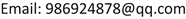Short-Term Traffic Flow Prediction Based on XGBoost

Wei Liu1, Subhash C. Bagui2, Hong’en Jia1

1College of Mathematics, Taiyuan University of Technology, Jinzhong Shanxi

2Department of Mathematics and Statistics, The University of West Florida, Pensacola, FL, USAReceived: Aug. 16th, 2020; accepted: Sep. 1st, 2020; published: Sep. 8th, 2020ABSTRACT

For short-term traffic flow prediction, in order to complete real-time accurate prediction, an extreme gradient boosting (XGBoost) short-term traffic flow prediction model based on Huber loss is established. By analyzing the periodicity and relevance of traffic flow data, time features are extracted and feature importance analysis is performed. Using this model and the extracted features for traffic flow prediction, the experimental results show that the model is superior to the extreme gradient boosting model based on mean square error loss and the extreme gradient boosting model based on average absolute error loss. At the same time, the model has higher prediction accuracy than gradient boosting regression model and support vector machine regression model, each error index is small, and the model training time is short, which meets the timeliness required by short-term traffic flow prediction.

Keywords:Traffic Flow Prediction, Extreme Gradient Boosting (XGBoost), Huber Loss Function, Feature Importance Analysis1. 引言

2. XGBoost原理

2.1. XGBoost目标函数定义

$\left\{\begin{array}{l}{\stackrel{^}{y}}_{i}^{\left(0\right)}=0\\ {\stackrel{^}{y}}_{i}^{\left(1\right)}={f}_{1}\left({x}_{i}\right)={\stackrel{^}{y}}_{i}^{\left(0\right)}+{f}_{1}\left({x}_{i}\right)\\ {\stackrel{^}{y}}_{i}^{\left(2\right)}={f}_{1}\left({x}_{i}\right)+{f}_{2}\left({x}_{i}\right)={\stackrel{^}{y}}_{i}^{\left(1\right)}+{f}_{2}\left({x}_{i}\right)\\ \text{\hspace{0.17em}}\text{ }\text{ }⋮\\ {\stackrel{^}{y}}_{i}^{\left(t\right)}=\underset{k=1}{\overset{t}{\sum }}{f}_{k}\left({x}_{i}\right)={\stackrel{^}{y}}_{i}^{\left(t-1\right)}+{f}_{t}\left({x}_{i}\right)\end{array}$ (1)

$\Omega \left({f}_{t}\right)=\gamma T+\frac{1}{2}\lambda \underset{j=1}{\overset{T}{\sum }}{\omega }_{j}^{2}$ (2)

$\begin{array}{c}Ob{j}^{\left(t\right)}=\underset{i=1}{\overset{n}{\sum }}l\left({y}_{i},{\stackrel{^}{y}}_{i}^{\left(t\right)}\right)+\underset{k=1}{\overset{t}{\sum }}\Omega \left({f}_{k}\right)\\ =\underset{i=1}{\overset{n}{\sum }}l\left({y}_{i},{\stackrel{^}{y}}_{i}^{\left(t-1\right)}+{f}_{t}\left({x}_{i}\right)\right)+\Omega \left({f}_{t}\right)+\text{constant}\end{array}$ (3)

$f\left(x+\Delta x\right)\approx f\left(x\right)+{f}^{\prime }\left(x\right)\Delta x+\frac{1}{2}{f}^{″}\left(x\right)\Delta {x}^{2}$ (4)

$\Delta x={f}_{t}\left({x}_{i}\right)$ (5)

$f\left(x\right)=l\left({y}_{i},{\stackrel{^}{y}}_{i}^{\left(t-1\right)}\right)$ (6)

${g}_{i}=\frac{\partial l\left({y}_{i},{\stackrel{^}{y}}_{i}^{\left(t-1\right)}\right)}{\partial {\stackrel{^}{y}}_{i}^{\left(t-1\right)}}$ (7)

${h}_{i}=\frac{{\partial }^{2}l\left({y}_{i},{\stackrel{^}{y}}_{i}^{\left(t-1\right)}\right)}{\partial {\stackrel{^}{y}}_{i}^{\left(t-1\right)}{}^{2}}$ (8)

$Ob{j}^{\left(t\right)}\approx \underset{i=1}{\overset{n}{\sum }}\left[l\left({y}_{i},{\stackrel{^}{y}}_{i}^{\left(t-1\right)}\right)+{g}_{i}{f}_{t}\left({x}_{i}\right)+\frac{1}{2}{h}_{i}{f}_{t}^{2}\left({x}_{i}\right)\right]+\Omega \left({f}_{t}\right)+\text{constant}$ (9)

$Ob{j}^{\left(t\right)}\approx \underset{i=1}{\overset{n}{\sum }}\left[{g}_{i}{f}_{t}\left({x}_{i}\right)+\frac{1}{2}{h}_{i}{f}_{t}^{2}\left({x}_{i}\right)\right]+\Omega \left({f}_{t}\right)$ (10)

2.2. XGBoost的目标函数求解

$\begin{array}{c}Ob{j}^{\left(t\right)}=\underset{i=1}{\overset{n}{\sum }}\left[{g}_{i}{f}_{t}\left({x}_{i}\right)+\frac{1}{2}{h}_{i}{f}_{t}^{2}\left({x}_{i}\right)\right]+\Omega \left({f}_{t}\right)\\ =\underset{i=1}{\overset{n}{\sum }}\left[{g}_{i}{w}_{q}\left({x}_{i}\right)+\frac{1}{2}{h}_{i}{\omega }_{q}^{2}\left({x}_{i}\right)\right]+\gamma T+\frac{1}{2}\lambda \underset{j=1}{\overset{T}{\sum }}{\omega }_{j}^{2}\\ =\underset{j=1}{\overset{T}{\sum }}\left[\left(\underset{i\in {I}_{j}}{\sum }{g}_{i}\right){w}_{j}+\frac{1}{2}\left(\underset{i\in {I}_{j}}{\sum }{h}_{i}+\lambda \right){\omega }_{j}^{2}\right]+\gamma T\\ =\underset{j=1}{\overset{T}{\sum }}\left[{G}_{j}{w}_{j}+\frac{1}{2}\left({H}_{j}+\lambda \right){\omega }_{j}^{2}\right]+\gamma T\end{array}$ (11)

${\omega }_{j}^{*}=-\frac{{G}_{j}}{{H}_{j}+\lambda }$ (12)

$Ob{j}^{*}=-\frac{1}{2}\underset{j=1}{\overset{T}{\sum }}\frac{{G}_{j}^{2}}{{H}_{j}+\lambda }+\gamma T$ (13)

$Gain=\frac{1}{2}\left[\frac{{G}_{L}^{2}}{{H}_{L}+\lambda }+\frac{{G}_{R}^{2}}{{H}_{R}+\lambda }-\frac{{\left({G}_{L}+{G}_{R}\right)}^{2}}{{H}_{L}+{H}_{R}+\lambda }\right]-\gamma$ (14)

3. 基于XGBoost算法的交通流预测模型

3.1. 自定义XGBoost目标函数

${L}_{\delta }\left({y}_{i},{\stackrel{˜}{y}}_{i}\right)=\left\{\begin{array}{l}\frac{1}{2}{\left({y}_{i}-{\stackrel{˜}{y}}_{i}\right)}^{2},\text{\hspace{0.17em}}\text{\hspace{0.17em}}|{y}_{i}-{\stackrel{˜}{y}}_{i}|\le \delta \\ \delta |{y}_{i}-{\stackrel{˜}{y}}_{i}|-\frac{1}{2}{\delta }^{2},\text{\hspace{0.17em}}\text{\hspace{0.17em}}\text{otherwise}\end{array}$ (15)

Huber损失是不可导函数，所以在本文中用的是Huber损失的可导逼近形式(伪Huber损失函数作为目标函数)，其定义如下：

${L}_{\delta }\left(x\right)={\delta }^{2}\left(\sqrt{1+{\left(\frac{x}{\delta }\right)}^{2}}-1\right)$ (16)

$\frac{\partial }{\partial x}\left({\delta }^{2}\left(\sqrt{1+\frac{{x}^{2}}{{\delta }^{2}}}-1\right)\right)=\frac{x}{\sqrt{1+\frac{{x}^{2}}{{\delta }^{2}}}}$ (17)

$\frac{{\partial }^{2}}{\partial {x}^{2}}\left({\delta }^{2}\left(\sqrt{1+\frac{{x}^{2}}{{\delta }^{2}}}-1\right)\right)=\frac{1}{{\left(1+\frac{{x}^{2}}{{\delta }^{2}}\right)}^{\frac{3}{2}}}$ (18)

3.2. 交通流预测模型实现流程

4. 实例分析

4.1. 数据来源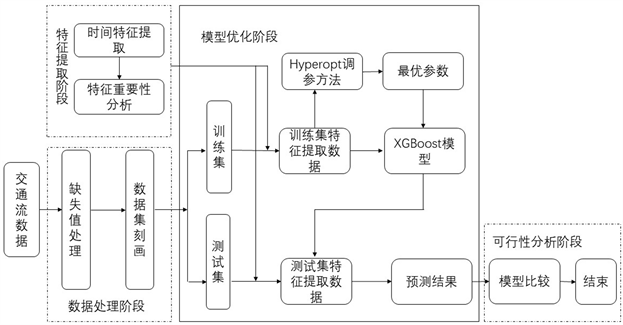Figure 1. Realization process of traffic flow prediction model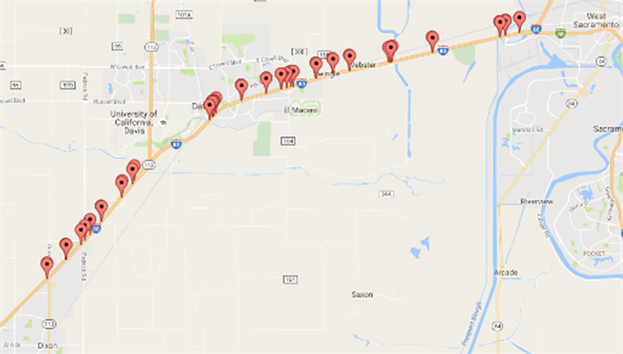Figure 2. Sensor settings in I80 Corridor, California

4.2. 数据预处理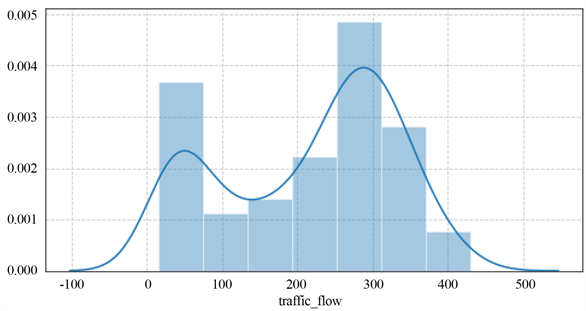Figure 3. One-day traffic flow data distribution map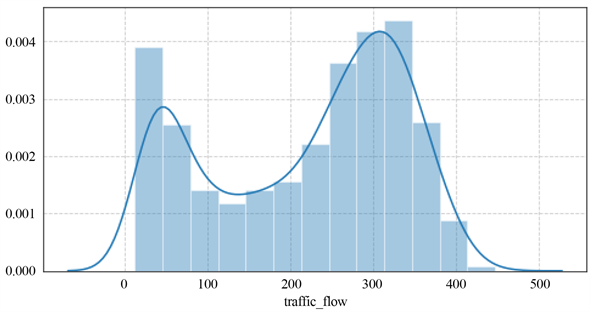Figure 4. One-week traffic flow data distribution map

4.3. 特征提取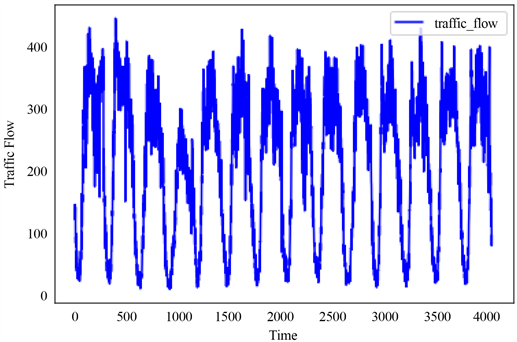Figure 5. Two-week traffic flow data graph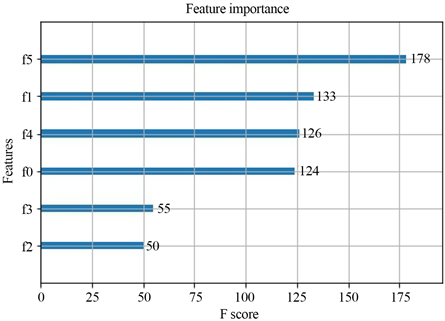Figure 6. Feature importance analysis chart

4.4. XGBoost参数调优

XGBoost模型参数众多，使用Hyperopt方法对各个参数进行调节，如表1所示。Table 1. Parameter value of traffic flow prediction model

1) n_estimators：弱学习器的最大迭代次数，或者说最大的弱学习器个数。n_estimators太小，容易欠拟合，n_estimators太大，又容易过拟合。

2) learning_rate：学习率，可以减少每一步的权重，提高模型的鲁棒性。

3) max_depth：数的最大深度。

4) min_child_weight：决定最小叶子节点样本权重和。

5) scale_pos_weight：样本十分不平衡时，将这个参数设置成正数，可以使算法更快收敛。

6) subsample：随机采样比例。

7) colsample_bytree：列采样率，也就是特征采样率。

8) gamma：分裂节点时，损失函数减小值只有大于等于gamma，节点才分裂。

4.5. 模型预测结果及分析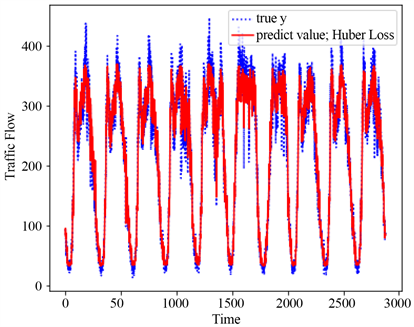Figure 7. Ten-day prediction graph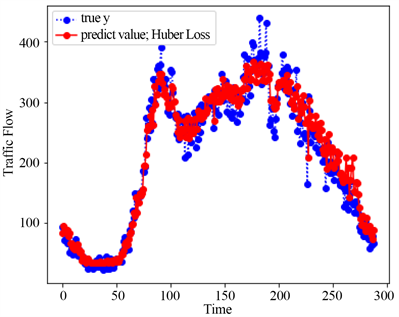Figure 8. Single-day prediction graph

5. 不同模型预测结果对比分析

5.1. 不同模型预测结果评价指标

$\text{RMSE}=\sqrt{\frac{1}{m}\underset{i=1}{\overset{m}{\sum }}{\left({y}_{i}-{\stackrel{^}{y}}_{i}\right)}^{2}}$ (19)

$\text{MAE}=\frac{1}{m}\underset{i=1}{\overset{m}{\sum }}|{y}_{i}-{\stackrel{^}{y}}_{i}|$ (20)

${R}^{2}=1-\frac{\underset{i=1}{\overset{m}{\sum }}{\left({y}_{i}-{\stackrel{^}{y}}_{i}\right)}^{2}}{\underset{i=1}{\overset{m}{\sum }}{\left({y}_{i}-{\stackrel{¯}{y}}_{i}\right)}^{2}}$ (21)

5.2. 不同模型预测结果对比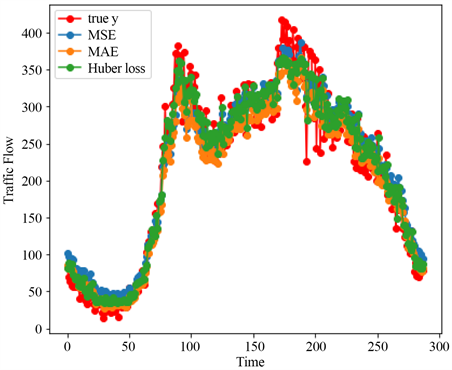Figure 9. Comparison of different objective functionsTable 2. Comparison of different objective functionsFigure 10. Comparison of different models

6. 结语

Short-Term Traffic Flow Prediction Based on XGBoost[J]. 应用数学进展, 2020, 09(09): 1364-1376. https://doi.org/10.12677/AAM.2020.99162

1. 1. 李敏, 黄迟. 集成学习下的短期交通流预测[J]. 济南大学学报(自然科学版), 2019, 33(5): 390-395.

2. 2. Ahmed, M.S. and Cook, A.R. (1979) Analysis of Freeway Traffic Time-Series Data by Using Box-Jenkins Technique. Transportation Research Board, 722, 1-9.

3. 3. Li, Y., Xiao, J., et al. (2016) Multiple Measures-Based Chaotic Time Series for Traffic Flow Prediction Based on Bayesian Theory. Nonlinear Dynamics, 85, 179-194. https://doi.org/10.1007/s11071-016-2677-5

4. 4. Wei, H., Cheng, Z., Sotelo, M.A., et al. (2017) Short-Term Vessel Traffic Flow Forecasting by Using an Improved Kalman Model. Cluster Computing, No. 10, 1-10.

5. 5. Guo, J.H. and Williams, B.M. (2010) Real-Time Short-Term Traffic Speed Level Forecasting and Uncertainty Quantification using Layered Kalman Filters. Transportation Research Record: Journal of the Transportation Research Board, 2175, 28-37. https://doi.org/10.3141/2175-04

6. 6. 李晓磊, 肖进丽, 刘明俊. 基于SARIMA模型的船舶交通流量预测研究[J]. 武汉理工大学学报(交通科学与工程版), 2017, 41(2): 329-332.

7. 7. 陆化普, 孙智源, 屈闻聪. 基于时空模型的交通流故障数据修正方法[J]. 交通运输工程学报, 2015, 15(6): 92-100.

8. 8. 钟颖, 邵毅明, 吴文文. 基于XGBoost的短时交通流预测模型[J]. 科学技术与工程, 2019, 19(30): 338-342.

9. 9. 苏美红, 张海. 基于不同损失函数的模型选择和正则化学习方法[J]. 纺织高校基础科学学报, 2014, 27(4): 464.

10. 10. 叶景, 李丽娟, 唐臻旭. 基于CNN-XGBoost的短时交通流预测[J]. 计算机工程与设计, 2020, 41(4): 1081-1086.